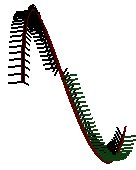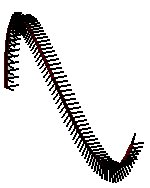# problem Frenet frame?

I just searched for Frenet frame and I got a Norway website. I don’t understand its language but I can know its code.

http://www.ia.hiof.no/~borres/gb/ma-frames/p-frames.html

But there’s a point I don’t undestand,

that if use the fomula below

T=C’
B=T x C’’
N=T x B

then Frenet frame look like this picture:but If use the fomula

T=C’
B=T x V
N=T x B

with V=(0.0 , 0.0 , 1.0 , 0.0) then frenet frame would change like this pictureI don’t understand. Please explain to meHi,…
The problem with the first formula is that the 2nd derivative (i.e. C’’) for the Bezier curve changes direction.

The second formula just takes a wild/educated guess at what C’’ might beYou might also have a look at http://en.wikipedia.org/wiki/Derivative if you’re not to familiar with derivatives.